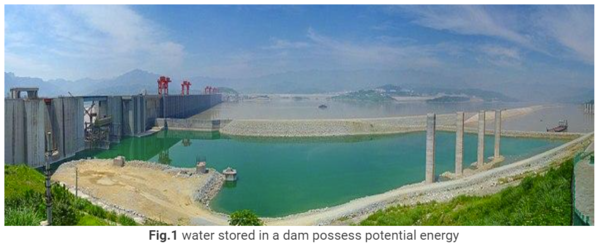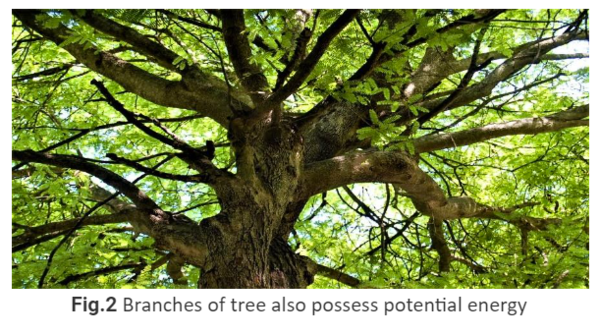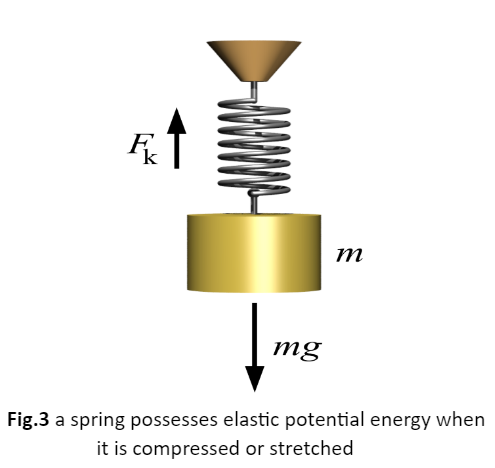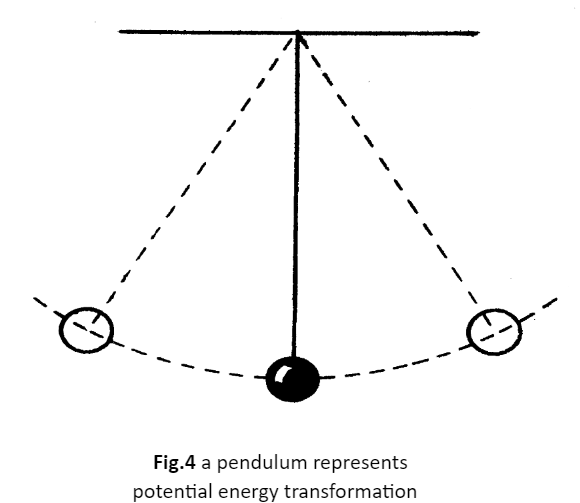• ### Browse All Lessons

##### Assign Lesson

Help Teaching subscribers can assign lessons to their students to review online!

 Tweet# Potential Energy

This lesson aligns with Next Generation Science Standards (NGSS) PS2.A

Introduction
Energy is the capacity to perform any physical activity or cause change. Energy is a fundamental term that we often use in our daily activities. Different forms of energy can be grouped into two general forms that are kinetic energy and potential energy. Some objects possess energy when moving and some objects have stored energy because of their position or shape. For example, a heavy ball of a demolished machine can store energy when it is held at an uplift position. In this article, we will learn about potential energy, the unit of potential energy, and types of potential energy.

Potential Energy
The energy that is stored in an object or substance is referred to as potential energy. This stored energy is based on the position, shape, or state of the object. In the 19th century, the term potential energy was introduced by Scottish engineer and physicist William Rankine. When the object is at rest, it also possesses potential energy.

For example, the water stored in dam reservoirs is used for different purposes such as generating electricity in hydropower plants, flood control, agriculture use, etc. The water kept in reservoirs is at rest and possesses some amount of potential energy. As we mentioned earlier, the object has potential energy when it is in a rest state.The stones sitting on an edge of a cliff and branches high up the tree also possess potential energy.Potential Energy Unit
The potential energy is measured in joule or $kgm^2//s^2$.

Types of Potential Energy
Potential energy can be categorized into main two types that are gravitational potential energy and elastic potential energy.

Gravitational Potential EnergyThe potential energy that is associated with an object’s height is known as gravitational potential energy. To better understand, let us take an object of mass m. We want to lift the object from the ground at a certain height h, the force is required to raise it. As we raised the object against gravity, we do work on it. When the work is done, we transfer energy to the object, that is stored in it. This stored energy due to its position is referred to as gravitational potential energy.

Gravitational potential energy = $weight xx height$
Gravitational potential energy = $mgh$

Effect of Weight and Height
The gravitational potential energy of an object depends on its weight and its height relative to the reference point. The object having more weight, possesses more gravitational energy. Similarly, the greater the height of the object, the greater its gravitational potential energy.

Elastic Potential Energy
Another type of potential energy is elastic potential energy which is stored in an object when it is squeezed or compressed. For example, a spring is hanging vertically from a ceiling. If some amount of force is applied on the other end in the up-direction, the spring will be compressed, as shown in the figure. The energy stored in the spring is in the form of elastic potential energy.Potential Energy Transformation
Potential energy can be transferred from one form to another form of energy and can transfer between objects.  For instance, have a look at a pendulum clock. When the bob of a pendulum is held at one point, it has the maximum potential energy due to its position. As we leave the bob, the potential energy is now converted into kinetic energy until it reaches another highest point. At this extreme position, all the kinetic energy will be converted again into potential energy. This process will repeat again and again until the pendulum stops. This example demonstrates that energy neither be created nor destroyed, it is only altered from one form to another.Summary
• The object possesses energy due to its height is referred to as potential energy that is measured in joule.
• Potential energy can be categorized into two types gravitational potential energy and elastic potential energy.
• The potential energy stored due to the height of an object is known as gravitational potential energy. For example, stones resting on the edge of a cliff.
• The potential energy stored in an object that can be stretched or compressed is known as elastic potential energy.
• Potential energy can be transformed from one form to another but can never be destroyed.

Related Worksheets: